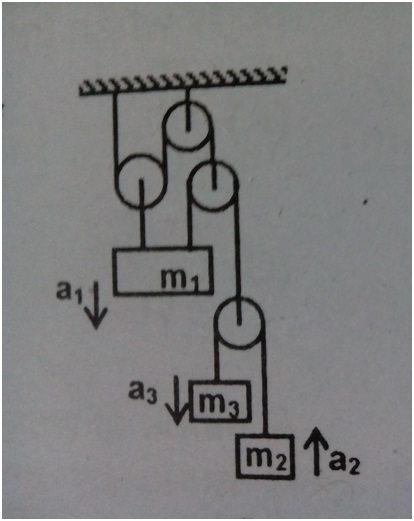# The pulleys moveThe pulley block system is shown in the figure. All strings and pulleys are ideal. The relation between $a_{1},a_{2} , a_{3}$ is given as $xa_{1}+ya_{2}+za_{3}=0$ where $x,y,z$ are co prime integers. Find $|x+y+z|$.

×